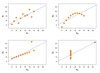Correlation coefficient

• 在二元变量的相关分析过程中比较常用的有Pearson相关系数，Spearman秩相关系数和判定系数。 Pearson相关系数 一般用于分析两个连续性变量之间的关系，其计算公式如下。 r=∑i=1n(xi−x‾)(yi−y‾)∑i=1n(xi−x‾)2...
数据探索
计算相关系数
为了更加准确地描述变量之间的线性相关程度，可以通过计算相关系统来进行相关分析。
在二元变量的相关分析过程中比较常用的有Pearson相关系数，Spearman秩相关系数和判定系数。
皮尔逊相关系数（Pearson Correlation Coefficient）
一般用于分析两个连续性变量之间的关系，其计算公式如下。
$r = { \sum_{i=1}^{n}(xi-\overline{x})(yi-\overline{y})\over\sqrt{\sum_{i=1}^{n}(xi-\overline{x})^2\sum_{i=1}^{n}(yi-\overline{y})^2}}$
相关系数r的取值范围：-1 <=  r <= 1
$\begin{cases} r > 0 为正相关，r<0为负相关\\ |r| = 0 表示不存在线性关系\\ |r| = 1 表示完全线性相关 \end{cases}$
0<|r|<1表示存在不同程度线性相关
$\begin{cases} |r|<=0.3为不存在线性相关\\ 0.3<|r|<=0.5为低度线性相关\\ 0.5<|r|<=0.8为显著线性相关\\ |r|>0.8为高度线性相关 \end{cases}$
Spearman秩相关系数
Pearson线性相关系数要求连续变量的取值服从正太分布。不服从正态分布的变量、分类或等级变量之间的关联性可采用Spearman秩相关系数，也称等级相关系数来描述。
其计算公式如下：
$r={1-{{6\sum_{i=1}^{n}(Ri-Qi)^2}\over{n(n^2-1)}}}$
研究表明，在正态分布假设下，Spearman秩相关系数与Pearson相关系数在效率上是等价的，而对于连续测量数据，更适合用Pearson相关系数来进行分析。
判定系数
判定系数是相关系数的平方，用$r^2$表示；用来衡量回归方程对y的解释程度。
判定系数取值范围：0<=$r^2$<=1, $r^2$越接近于1，表示x与y之间的相关性越强；
$r^2​$越接近于0，表明两个变量之间几乎没有直线相关关系。


展开全文数据 数据分析
• 在二元变量的相关分析过程中比较常用的有Pearson相关系数，Spearman秩相关系数和判定系数。 皮尔逊相关系数（Pearson Correlation Coefficient） 一般用于分析两个连续性变量之间的关系，其计算公式如下。 r=∑i=1n...
数据探索
计算相关系数
为了更加准确地描述变量之间的线性相关程度，可以通过计算相关系统来进行相关分析。
在二元变量的相关分析过程中比较常用的有Pearson相关系数，Spearman秩相关系数和判定系数。
皮尔逊相关系数（Pearson Correlation Coefficient）
一般用于分析两个连续性变量之间的关系，其计算公式如下。
$r = { \sum_{i=1}^{n}(xi-\overline{x})(yi-\overline{y})\over\sqrt{\sum_{i=1}^{n}(xi-\overline{x})^2\sum_{i=1}^{n}(yi-\overline{y})^2}}$
相关系数r的取值范围：-1 <=  r <= 1
$\begin{cases} r > 0 为正相关，r<0为负相关\\ |r| = 0 表示不存在线性关系\\ |r| = 1 表示完全线性相关 \end{cases}$
0<|r|<1表示存在不同程度线性相关
$\begin{cases} |r|<=0.3为不存在线性相关\\ 0.3<|r|<=0.5为低度线性相关\\ 0.5<|r|<=0.8为显著线性相关\\ |r|>0.8为高度线性相关 \end{cases}$
近似计算公式
​		上面的公式有一个问题在于算法时可能需要对数据进行多遍扫描。幸运的是，对于算法实现人员而言，还有一个皮尔逊相关系数的近似计算公式：
$r=\frac{\sum_{i=1}^nx_iy_i-\frac{\sum_{i=1}^nx_i\sum_{i=1}^ny_i}{n}}{\sqrt{\sum_{i=1}^nx_i^2-\frac{(\sum_{i=1}^nx_i)^2}{n}}\sqrt{\sum_{i=1}^ny_i^2-\frac{(\sum_{i=1}^ny_i)^2}{n}}}$
Python
def pearson(rating1, rating2):
sum_xy = 0
sum_x = 0
sum_y = 0
sum_x2 = 0
sum_y2 = 0
n = 0
for key in rating1:
if key in rating2:
n += 1
x = rating1[key]
y = rating2[key]
sum_xy += x * y
sum_x += x
sum_y += y
sum_x2 += pow(x, 2)
sum_y2 += pow(y, 2)
# now compute denominator
denominator = sqrt(sum_x2 - pow(sum_x, 2) / n) * sqrt(sum_y2 - pow(sum_y, 2) / n)
if denominator == 0:
return 0
else:
return (sum_xy - (sum_x * sum_y) / n) / denominator


Spearman秩相关系数
Pearson线性相关系数要求连续变量的取值服从正太分布。不服从正态分布的变量、分类或等级变量之间的关联性可采用Spearman秩相关系数，也称等级相关系数来描述。
其计算公式如下：
$r={1-{{6\sum_{i=1}^{n}(Ri-Qi)^2}\over{n(n^2-1)}}}$
研究表明，在正态分布假设下，Spearman秩相关系数与Pearson相关系数在效率上是等价的，而对于连续测量数据，更适合用Pearson相关系数来进行分析。
判定系数
判定系数是相关系数的平方，用$r^2$表示；用来衡量回归方程对y的解释程度。
判定系数取值范围：0<=$r^2$<=1, $r^2$越接近于1，表示x与y之间的相关性越强；
$r^2$越接近于0，表明两个变量之间几乎没有直线相关关系。
相似度的选择
如果数据稠密（几乎所有属性都没有零值）且属性值大小十分重要，那么使用诸如欧式距离或者曼哈顿距离。
如果数据稀疏，考虑使用余弦相似度。
相关代码
import codecs
from math import sqrt

users = {"Angelica": {"Blues Traveler": 3.5, "Broken Bells": 2.0,
"Norah Jones": 4.5, "Phoenix": 5.0,
"Slightly Stoopid": 1.5,
"The Strokes": 2.5, "Vampire Weekend": 2.0},

"Bill":{"Blues Traveler": 2.0, "Broken Bells": 3.5,
"Slightly Stoopid": 3.5, "Vampire Weekend": 3.0},

"Chan": {"Blues Traveler": 5.0, "Broken Bells": 1.0,
"Deadmau5": 1.0, "Norah Jones": 3.0, "Phoenix": 5,
"Slightly Stoopid": 1.0},

"Dan": {"Blues Traveler": 3.0, "Broken Bells": 4.0,
"Slightly Stoopid": 4.5, "The Strokes": 4.0,
"Vampire Weekend": 2.0},

"Hailey": {"Broken Bells": 4.0, "Deadmau5": 1.0,
"Norah Jones": 4.0, "The Strokes": 4.0,
"Vampire Weekend": 1.0},

"Jordyn":  {"Broken Bells": 4.5, "Deadmau5": 4.0,
"Norah Jones": 5.0, "Phoenix": 5.0,
"Slightly Stoopid": 4.5, "The Strokes": 4.0,
"Vampire Weekend": 4.0},

"Sam": {"Blues Traveler": 5.0, "Broken Bells": 2.0,
"Norah Jones": 3.0, "Phoenix": 5.0,
"Slightly Stoopid": 4.0, "The Strokes": 5.0},

"Veronica": {"Blues Traveler": 3.0, "Norah Jones": 5.0,
"Phoenix": 4.0, "Slightly Stoopid": 2.5,
"The Strokes": 3.0}
}

class recommender:

def __init__(self, data, k=1, metric='pearson', n=5):
""" initialize recommender
currently, if data is dictionary the recommender is initialized
to it.
For all other data types of data, no initialization occurs
k is the k value for k nearest neighbor
metric is which distance formula to use
n is the maximum number of recommendations to make"""
self.k = k
self.n = n
self.userid2name = {}
self.productid2name = {}
# for some reason I want to save the name of the metric
self.metric = metric
if self.metric == 'pearson':
self.fn = self.pearson
#
# if data is dictionary set recommender data to it
#
if type(data).__name__ == 'dict':
self.data = data

def convertProductID2name(self, id):
"""Given product id number return product name"""
if id in self.productid2name:
return self.productid2name[id]
else:
return id

def userRatings(self, id, n):
"""Return n top ratings for user with id"""
print ("Ratings for " + self.userid2name[id])
ratings = self.data[id]
print(len(ratings))
ratings = list(ratings.items())
ratings = [(self.convertProductID2name(k), v)
for (k, v) in ratings]
# finally sort and return
ratings.sort(key=lambda artistTuple: artistTuple,
reverse = True)
ratings = ratings[:n]
for rating in ratings:
print("%s\t%i" % (rating, rating))

"""loads the BX book dataset. Path is where the BX files are
located"""
self.data = {}
i = 0
#
# First load book ratings into self.data
#
f = codecs.open(path + "BX-Book-Ratings.csv", 'r', 'utf8')
for line in f:
i += 1
#separate line into fields
fields = line.split(';')
user = fields.strip('"')
book = fields.strip('"')
rating = int(fields.strip().strip('"'))
if user in self.data:
currentRatings = self.data[user]
else:
currentRatings = {}
currentRatings[book] = rating
self.data[user] = currentRatings
f.close()
#
# Now load books into self.productid2name
# Books contains isbn, title, and author among other fields
#
f = codecs.open(path + "BX-Books.csv", 'r', 'utf8')
for line in f:
i += 1
#separate line into fields
fields = line.split(';')
isbn = fields.strip('"')
title = fields.strip('"')
author = fields.strip().strip('"')
title = title + ' by ' + author
self.productid2name[isbn] = title
f.close()
#
#  Now load user info into both self.userid2name and
#
f = codecs.open(path + "BX-Users.csv", 'r', 'utf8')
for line in f:
i += 1
#print(line)
#separate line into fields
fields = line.split(';')
userid = fields.strip('"')
location = fields.strip('"')
if len(fields) > 3:
age = fields.strip().strip('"')
else:
age = 'NULL'
if age != 'NULL':
value = location + '  (age: ' + age + ')'
else:
value = location
self.userid2name[userid] = value
f.close()
print(i)

def pearson(self, rating1, rating2):
sum_xy = 0
sum_x = 0
sum_y = 0
sum_x2 = 0
sum_y2 = 0
n = 0
for key in rating1:
if key in rating2:
n += 1
x = rating1[key]
y = rating2[key]
sum_xy += x * y
sum_x += x
sum_y += y
sum_x2 += pow(x, 2)
sum_y2 += pow(y, 2)
if n == 0:
return 0
# now compute denominator
denominator = (sqrt(sum_x2 - pow(sum_x, 2) / n)
* sqrt(sum_y2 - pow(sum_y, 2) / n))
if denominator == 0:
return 0
else:
return (sum_xy - (sum_x * sum_y) / n) / denominator

"""creates a sorted list of users based on their distance to
distances = []
for instance in self.data:
self.data[instance])
distances.append((instance, distance))
# sort based on distance -- closest first
distances.sort(key=lambda artistTuple: artistTuple,
reverse=True)
return distances

def recommend(self, user):
"""Give list of recommendations"""
recommendations = {}
# first get list of users  ordered by nearness
nearest = self.computeNearestNeighbor(user)
#
# now get the ratings for the user
#
userRatings = self.data[user]
#
# determine the total distance
totalDistance = 0.0
for i in range(self.k):
totalDistance += nearest[i]
# now iterate through the k nearest neighbors
# accumulating their ratings
for i in range(self.k):
# compute slice of pie
weight = nearest[i] / totalDistance
# get the name of the person
name = nearest[i]
# get the ratings for this person
neighborRatings = self.data[name]
# get the name of the person
# now find bands neighbor rated that user didn't
for artist in neighborRatings:
if not artist in userRatings:
if artist not in recommendations:
recommendations[artist] = (neighborRatings[artist]
* weight)
else:
recommendations[artist] = (recommendations[artist]
+ neighborRatings[artist]
* weight)
# now make list from dictionary
recommendations = list(recommendations.items())
recommendations = [(self.convertProductID2name(k), v)
for (k, v) in recommendations]
# finally sort and return
recommendations.sort(key=lambda artistTuple: artistTuple,
reverse = True)
# Return the first n items
return recommendations[:self.n]




展开全文• 参考维基百科 https://zh.wikipedia.org/wiki/%E7%9A%AE%E5%B0%94%E9%80%8A%E7%A7%AF%E7%9F%A9%E7%9B%B8%E5%85%B3%E7%B3%BB%E6%95%B0 百度百科 ...
参考材料：
维基百科 https://zh.wikipedia.org/wiki/%E7%9A%AE%E5%B0%94%E9%80%8A%E7%A7%AF%E7%9F%A9%E7%9B%B8%E5%85%B3%E7%B3%BB%E6%95%B0

今天老板突然推荐了解下皮尔森相关系数，有些莫名其妙，看了下，就是之前在数理统计里学到的相关系数，还是比较容易理解的，不过还是写点记录下学到的东西吧。

皮尔森相关系数（Pearson correlation coefficient）也称皮尔森积矩相关系数(Pearson product-moment correlation coefficient) ，是一种线性相关系数，是最常用的一种相关系数。记为r，用来反映两个变量X和Y的线性相关程度，r值介于-1到1之间，绝对值越大表明相关性越强。

定义：

总体相关系数ρ定义为两个变量X、Y之间的协方差和标准差的比值，如下：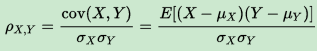估算样本的协方差和标准差，可得到样本相关系数（即样本皮尔森相关系数），常用r表示：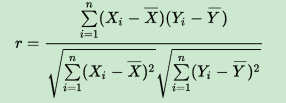r还可以由(Xi,Yi)样本点的标准分数均值估计得到与上式等价的表达式：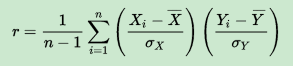其中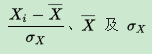为Xi样本的标准分数、样本均值和样本标准差，n为样本数量。

物理意义：

皮尔森相关系数反映了两个变量的线性相关性的强弱程度，r的绝对值越大说明相关性越强。当r>0时，表明两个变量正相关，即一个变量值越大则另一个变量值也会越大；当r<0时，表明两个变量负相关，即一个变量值越大则另一个变量值反而会越小；当r=0时，表明两个变量不是线性相关的（注意只是非线性相关），但是可能存在其他方式的相关性（比如曲线方式）；当r=1和-1时，意味着两个变量X和Y可以很好的由直线方程来描述，所有样本点都很好的落在一条直线上。

皮尔森距离：

通过皮尔森系数定义：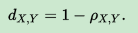皮尔森系数范围为[-1,1]，因此皮尔森距离范围为[0,2]。
展开全文统计学
• Pearson相关性系数可以看出是升级版的欧氏距离平方，因为它提供了对于变量取值范围不同的处理步骤。因此对不同变量间的取值范围没有要求（unit free）,最后得到的相关性所衡量的是趋势，而不同变量量纲上差别在计算...
Pearson相关性系数可以看出是升级版的欧氏距离平方，因为它提供了对于变量取值范围不同的处理步骤。因此对不同变量间的取值范围没有要求（unit free）,最后得到的相关性所衡量的是趋势，而不同变量量纲上差别在计算过程中去掉了，等价于z-score标准化。
而未经升级的欧式距离以及cosine相似度，对变量的取值范围是敏感的，在使用前需要进行适当的处理。在低维度可以优先使用标准化后的欧式距离或者其他距离度量，在高维度时Pearson相关系数更加适合。
欧氏距离（Euclidean Distance）是常见的相似性度量方法，可求两个向量间的距离，取值范围为0至正无穷。显然，如果两个向量间的距离较小，那么向量也肯定更为相似。此处需要注意的一点是，欧氏距离计算默认对于每一个维度给予相同的权重，因此如果不同维度的取值范围差别很大，那么结果很容易被某个维度所决定。解决方法除了对数据进行处理以外，还可以使用加权欧氏距离，不同维度使用不同的权重。
公式1：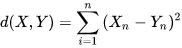Pearson相关性系数（Pearson Correlation）是衡量向量相似度的一种方法。输出范围为-1到+1, 0代表无相关性，负值为负相关，正值为正相关。
公式2：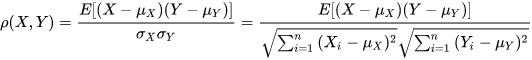Cosine相似度也是一种相似性度量，输出范围和Pearson相关性系数一致，含义也相似。
公式3：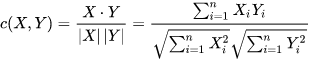标准化（Standardization）是一种常见的数据缩放手段，标准化后的数据均值为0，标准差为1。
公式4：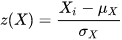平方和（Summed Square）与样本方差（Sample Variance）之间的关系：
公式5：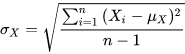公式6：由公式5可得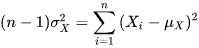展开全文• 概念：Pearson相关系数 （Pearson CorrelationCoefficient）是用来衡量两个数据集合是否在一条线上面，它用来衡量定距变量间的线性关系。 注： 【定距变量】 若想理解定距变量，需要与其他变量...矩阵
• 皮尔森相关系数为了确定 每个特征之间是否紧密相关，如果很相关就属于重复特征，可以去除。 我们输入机器学习模型中的每个特征都独一无二，这才是最佳。 python实现代码 import seaborn as sns #图表模块 import ...机器学习 特征工程
• 一、相关系数： ...二、Pearson相关系数： 1. 先给出公式推导： ①首先由Pearson相关系数的定义可知， ②这里，分子cov表示协方差，分母表示标准差（以两个变量为例）： 这里分母位...
• 统计相关系数简介     由于使用的统计相关系数比较频繁，所以这里就利用几篇文章简单介绍一下这些系数。   相关系数：考察两个事物（在数据里我们称之为变量）之间的相关程度。   如果有两个变量：X、Y，...相关分析 相关系数
• 1、Pearson皮尔森相关系数皮尔森相关系数也叫皮尔森积差相关系数，用来反映两个变量之间相似程度的统计量。或者说用来表示两个向量的相似度。皮尔森相关系数计算公式如下：　分子是协方差，分母两个向量的标准差的...
• SLNum=iris(:,1); SWNum=iris(:,2); PLNum=iris(:,3); PWNum=iris(:,4); Num=[SLNum,SWNum,PLNum,PWNum]; coeff1=corr(SLNum,SWNum,'type','Spearman'); coeff2=corr(SLNum,PLNum,'type','Spearman');...c...
• 统计学的相关系数经常使用的有三种:皮尔森（pearson相关系数和斯皮尔曼（spearman）相关系数和肯德尔（kendall）相关系数.皮尔森相关系数是衡量线性关联性的程度，p的一个几何解释是其代表两个变量的取值根据均值...
• import pandas as pd df = pd.read_csv('demo.csv') ...df.corr() #计算pearson相关系数 df.corr('kendall') #计算kendall相关系数 df.corr('spearman') #计算spearman相关系数 相关系数如下图所示： ...
• 三个相关性系数pearson, spearman, kendall）反应的都是两个变量之间变化趋势的方向以及程度，其值范围为-1到+1，0表示两个变量不相关，正值表示正相关，负值表示负相关，值越大表示相关性越强。 1. person ...
• 分子是两个变量的协方差， 分母是两个变量标准差乘积。... # 计算分子，协方差————按照协方差公式，本来要除以n的，由于在相关系数中上下同时约去了n，于是可以不除以n cov_ab = sum([(x - a_avg)*(y - ...
• ## Pearson相关系数

千次阅读 2019-10-01 20:55:11
（1）Pearson's r，称为皮尔逊相关系数Pearson correlation coefficient），用来反映两个随机变量之间的线性相关程度。 （2）pearson是一个介于 -1 和1 之间的值。 （3）当两个变量的线性关系增强时，相关系数...
• 计算积距pearson相关系数，连续性变量才可采用;计算Spearman秩相关系数，适合于定序变量或不满足正态分布假设的等间隔数据; 计算Kendall秩相关系数，适合于定序变量或不满足正态分布假设的等间隔数据。  计算相关...机器学习
• import numpy as np data = np.array([[10,10,8,9,7],[4,5,4,3,3],[3,3,1,1,1]]) data array([[10, 10, 8, 9, 7], [ 4, 5, 4, 3, 3], [ 3, 3, 1, 1, 1]]) ...#行与行之间的相关系数 np.corrcoef(da......